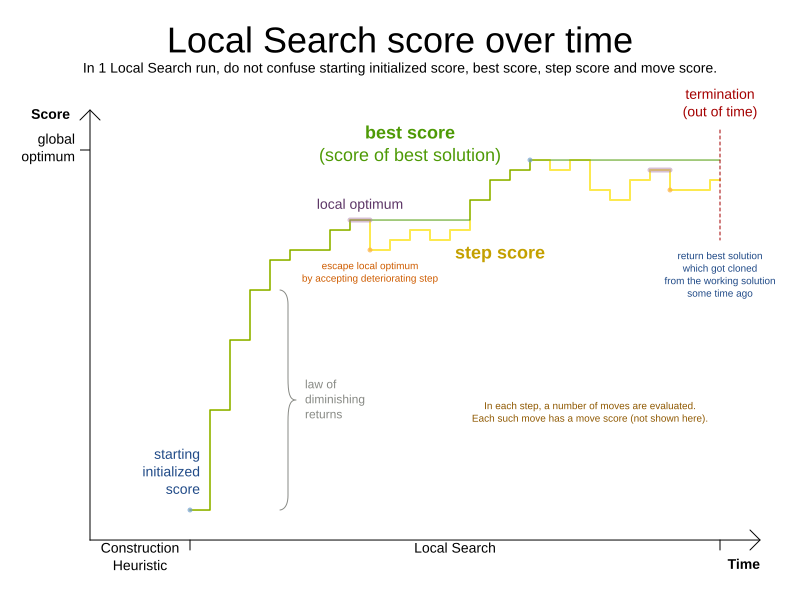# Discrete Optimization (Coursera)

2 minute read## Constraint programming

• Exact.
• Focus on feasibility
• Example problems: map coloring, n-queens, scheduling
• Libraries: OR-Tools (CP-SAT)1. Use constraints (instead of the objective) to reduce the (finite) domain of the variables (branch and prune).
2. Each type of constraint has dedicated algorithms that exploits its structure (feasibility test and pruning).
3. Make choices when can’t make more deductions (a fixpoint is reached).
4. Backtrack when the choice is wrong (domain of a variable is empty).
• Global constraints: `all-different`, `sum`, `element`, `cumulative`, `gcc`, etc. Discover infeasibilities earlier and prune the search space more.
• Redundant contraints: express properties not captured by the model (do not remove solutions but prune the search space).
• Reification: transform constraints into boolean variables.
• Channeling: if-then-else
• Symmetry breaking:
• variable symmetries: impose an (lexicographic) ordering on the variables
• value symmetries: sort variables `var[i] <= max(var[j] j in 0..i-1)`
• Heuristic method
• Graph exploration starting with an infeasible or suboptimal solution.
• Example problems: TSP, n-queens
• Libraries: NA
1. Generate a initial solution
2. Compute the neighbourhood (legal or not).
3. Select a new state from the neighbourhood and repeat.Heuristics: how to choose the next neighbour using local information, gets to a local minimum

• Best neighbour
• First neighbour
• Multi-stage: avoid scanning the whole neighbourhood (max/min-conflict, min-conflict).

Metaheuristics: how to escape the local minima using memory or learning

• Iterated Local Search (multistart, restart): run multiple times with different starting configurations.
• Metropolist Heuristic: allow degrading moves with some probability proportional to the degradation and a temperature.
• Simulated annealing: start with a high temperature (almost random walk) and decrease it progressively. You can also restart or reheat it.
• Tabu search: mark visited nodes (tabu list and tabu nodes) and avoid them. (Short-term memory, store transitions and objective values instead of states)

## Linear Programming

• Exact (simplex).
• No integer variables, only linear constraints and objective.
• Example problems: transportation, optimal diet
• Libraries: Pyomo, PuLP, CVXPY, GLOP• Convexity:
• Convex combinations:• Convex set: set that contains all the convex combinations of its points.
• Intersection of convex sets is convex
• Every point in a polytope is a convex combination of its vertices.
• At least one of the optimal solutions is a vertex (basic feasible solution).
• Primal simplex: primal always feasible, dual not (until the optimal).
• Dual simplex: dual always feasible, primal not. Useful when adding new constraints to the primal.

## Mixed Integer Programming

• Exact (branch and bound).
• Focus on the objective.
• Like linear programs but with integrality constraints.
• Example problems: knapsack, warehouse location, scheduling
• Libraries: Pyomo, PuLP, Python-mip, OR-Tools (CBC)

Branch and bound:

• Prune (bound) with (Linear) Relaxation
• Branch on variable with most fractional value (ceil and floor)
• Global view of all constraints
• Effective if linear relaxation is strong
• Good with many 0/1 variables

Big-M formulation example:

``````x != y

(x <= y-1) or (x >= y+1)

Add new binary b and a big number M:
x <= y - 1 + b*M
x >= y + 1 - (1-b)*M
``````

Tags:

Updated: